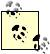# Figuring Out Required Rate of Return

Problem

You want to figure out the required rate of return for some given present value to grow to a desired future value.

Solution

Use the RATE function.

Discussion

Let's say you have \$5,000 now and want to know what rate of return would be required for that sum to grow to \$15,000 in 10 years if interest is compounded annually. The RATE function can figure this out for you.

The syntax for RATE is =RATE(nper, pmt, pv, fv, type, guess), where nper is the number of payment periods, pmt is the payment per period, pv is the present value, fv is the future value, type is an indicator of the payment type (0 for payments at the end of each pay period or 1 for payments at the beginning of each pay period), and guess is an initial guess at the required interest rate.RATE performs an iterative search for the required rate and your initial guess helps it converge. guess is actually an optional parameter and you can omit it if you'd like.

For our example, the formula =RATE(10, 0, -5000, 15000, 0, 0.1) returns a required interest rate of 11.61%. Notice that I entered the present value as a negative value, indicating an out payment. The signs for pv and fv must be opposite (fv can be 0); otherwise, RATE returns an error.

Take a look at the other recipes in this chapter to learn about other calculations of the time value of money.Excel Scientific and Engineering Cookbook (Cookbooks (OReilly))
ISBN: 0596008791
EAN: 2147483647
Year: N/A
Pages: 206
Authors: David M Bourg